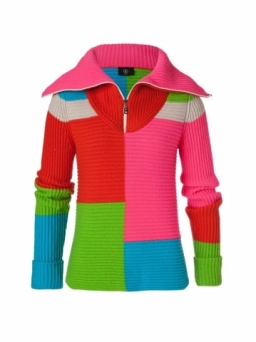# Danina's 7232

Mom bought Honza and Dana's jackets. She paid CZK 1,126 for them. How many crowns did Danina's jacket cost when it was 108 CZK cheaper than Honza's?

d =  509 Kc

### Step-by-step explanation:

1126 = d + (d+108)

2d = 1018

d = 1018/2 = 509

d = 509

Our simple equation calculator calculates it.Did you find an error or inaccuracy? Feel free to write us. Thank you!

Tips for related online calculators
Do you have a linear equation or system of equations and looking for its solution? Or do you have a quadratic equation?